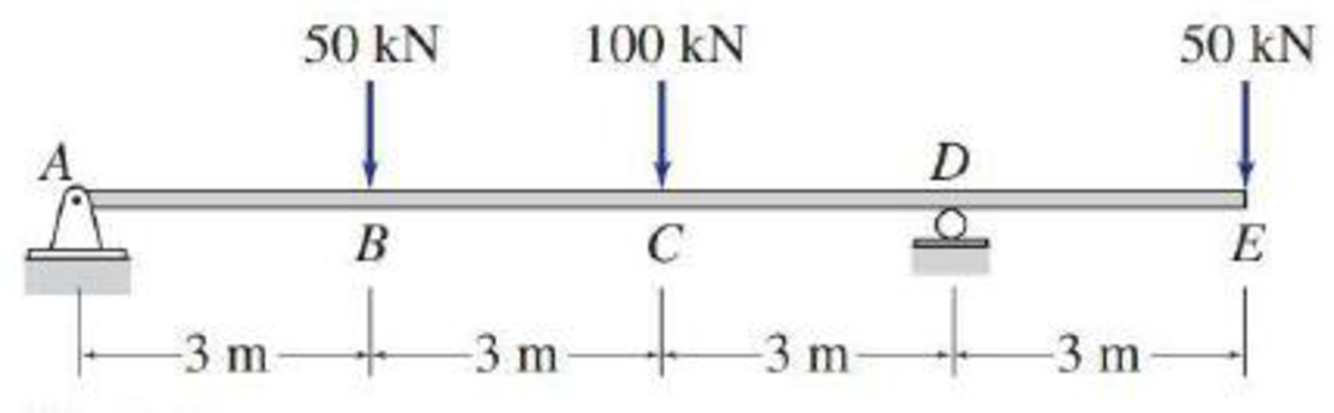# 5.29 through 5.51 Draw the shear and bending moment diagrams and the qualitative deflected shape for the beam shown.

#### Solutions

Chapter
Section
Chapter 5, Problem 32P
Textbook Problem
1 views

## 5.29 through 5.51 Draw the shear and bending moment diagrams and the qualitative deflected shape for the beam shown.To determine

Plot the shear and bending moment diagram and the qualitative deflected shape.

### Explanation of Solution

Sign conversion:

Apply the sign convention for calculating the equations of equilibrium as below.

• For the horizontal forces equilibrium condition, take the force acting towards right side as positive (+) and the force acting towards left side as negative ().
• For the vertical forces equilibrium condition, take the upward force as positive (+) and downward force as negative ().
• For moment equilibrium condition, take the clockwise moment as negative and counter clockwise moment as positive.

Apply the following sign convention for calculating the shear and bending moments.

• When the portion of the beam considered is left of the section, then the external force acting to the left are considered as positive.
• When the portion of the beam considered is right of the section, then the external force acting to the right are considered as positive.
• When the portion of the beam considered is left of the section, then the external force acting upward are considered as positive.
• When the portion of the beam considered is right of the section, then the external force acting downward are considered as positive.
• When the portion of the beam considered is left of the section, then the clockwise moments are considered as positive.
• When the portion of the beam considered is right of the section, then the counterclockwise moments are considered as positive.

Calculation:

Show the free-body diagram of the entire beam as in Figure 1.

Find the horizontal reaction at point A by resolving the horizontal equilibrium.

+Fx=0Ax=0Ax=0

Find the vertical reaction at point D by taking moment about point A.

+MA=050(3)100(6)+Dy(9)50(12)=0150600+9Dy600=0Dy=150kN

Find the vertical reaction at point A by resolving the vertical equilibrium.

+Fy=0Ay50100+Dy50=0Ay200+150=0Ay=50kN

Show the reaction of the beam as in Figure 2.

### Still sussing out bartleby?

Check out a sample textbook solution.

See a sample solution

#### The Solution to Your Study Problems

Bartleby provides explanations to thousands of textbook problems written by our experts, many with advanced degrees!

Get Started

Find more solutions based on key concepts
Fins, or extended surfaces, commonly are used in a variety of engineering applications to enhance cooling. Comm...

Engineering Fundamentals: An Introduction to Engineering (MindTap Course List)

What is an internet censorship?

Fundamentals of Information Systems

Determine the resultant of the forces shown.

International Edition---engineering Mechanics: Statics, 4th Edition

What might cause you to use diagnostic tools to scan a computer for hardware errors?

Enhanced Discovering Computers 2017 (Shelly Cashman Series) (MindTap Course List)

What is a correlated subquery? Give an example.

Database Systems: Design, Implementation, & Management

What is the purpose of a benchmark test? Suggest at least two examples of benchmarks.

Systems Analysis and Design (Shelly Cashman Series) (MindTap Course List)

Write a query to display the products that have a price greater than 50.

Database Systems: Design, Implementation, & Management

How is an incident response plan different from a disaster recovery plan?

Principles of Information Security (MindTap Course List)

List three types of screwdriver tips.

Precision Machining Technology (MindTap Course List)

If your motherboard supports ECC DDR3 memory, can you substitute non-ECC DDR3 memory?

A+ Guide to Hardware (Standalone Book) (MindTap Course List)